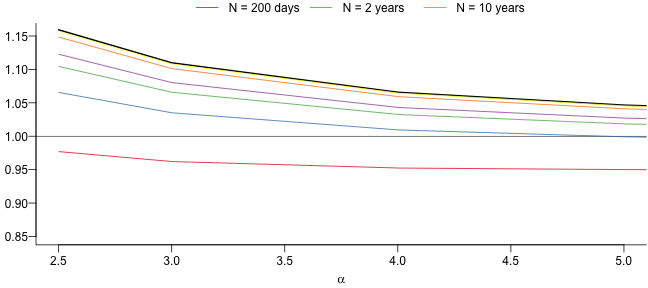# Why Risk is so Hard to Measure

### ES and VaR comparison plots

$\DeclareMathOperator{\VaR}{VaR}$ $\DeclareMathOperator{\ES}{ES}$ $\DeclareMathOperator{\E}{E}$

The plots below show the ratio of ES to VaR \begin{equation} \frac{\ES(p_1)}{\VaR(p_2)} \end{equation} in both the case where the probabilities are the same, 99% and where we have the Basel III probabilities of $p_2=99\%, p_1=97.5\%$.

The figures show everything from a very small sample size, 200 days to 50 years or 50*250 days. In the largest size, we have reached the asymtootics, but not for the smaller sizes. There is no point in plotting N=100 since then VaR and ES are the same.

The notable result is that as the sample size becomes smaller,
so does the ratio between ES and VaR and for the smaller sample
sizes, the ES 97.5% is smaller than VaR 99%.
Since we are more likely to encounter the small sample sizes in
practical use, a move to ES 97.5% from is likely to result in
lower market risk forecasts.

Is that what the Basel committee intended?

### 99% for both

#### Student-tThe results are as expected, as the sample size becomes large, we get back the theoretic value, and as the tail becomes thinner, the differences narrow.

#### ParetoThere is no qualitative difference between simulating from the Pareto, we get the same results as for the student to

### ES 97.5 and VaR 99

Here we look of the Basel III numbers, 97.5% ES compared to 99% VaR.

#### Student-tThe notable change here is that ES only retains a size advantage at the thickest tails, and for the smallest sample sizes the VaR may even be bigger,

#### ParetoThe Pareto yields the same qualitative results as the student